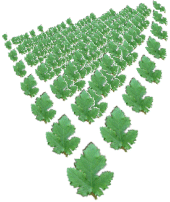Fractal plantations

The machine draws fractals of type IFS by the chaos game method. Additional, the IFS figure is transformed by a hierarchy transformation system. The results are interesting fractal plantations like the Sierpinsky-Crystal and the Fern-Sierpinsky. Choose a figure and a hierarchy system from the choices under the image. All of the transformation systems in the left choice could be combined with those of the right choice in order to create many different image types. The center checkbox decides whether the base figure is shown or not.

Hide All Points Show Figure IFS Group Points

The Fractal Plantations MachineMoving the circles which show the fixed points of the transformations (similiar to IFS II) will multiply image variations. If you drag a single circle to a different place (white for figure functions and yellow for hierarchy functions) you will change one function's behavior and therefor form a new figure. If you've checked "Connect" dragging one circle will move the whole figure or hierarchy system.

The factor initialized with different values is responsible for the relation between the use of the figure and the hierarchy transformation system. If you set the factor to "1" only the base figure will be drawn (you'll see nothing with base figure switched off!). Rising the factor will give the hierarchy system more influence.